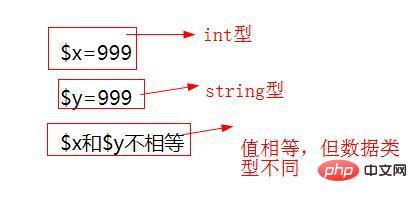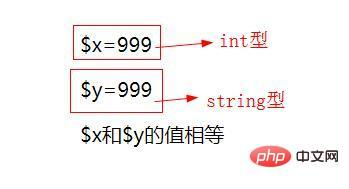# php中三个等号是什么意思===全等比较两个操作数的值是否相等，同时检测它们的类型是否相同

• 如果两个变量（表达式或常量）包含相同的值和相同的数据类型，则此运算符返回true，否则返回false。

```<?php
// 给变量赋整数值
\$x = 999;
echo '\$x='.\$x."<br>";
// 给变量赋字符串值
\$y = '999';
echo '\$y='.\$y."<br>";
//比较\$x 和\$y
if (\$x === \$y)
echo '\$x和\$y相等';
else
echo '\$x和\$y不相等';
?>``````<?php
// 给变量赋整数值
\$x = 999;
echo '\$x='.\$x."<br>";
// 给变量赋字符串值
\$y = '999';
echo '\$y='.\$y."<br>";
//比较\$x 和\$y
if (\$x == \$y)
echo '\$x和\$y的值相等';
else
echo '\$x和\$y的值不相等';
?>```\$a == \$b等于如果类型转换后 \$a 和 \$b 的值相等，则返回 TRUE，否则返回 FALSE
\$a === \$b全等如果 \$a 和 \$b 不仅值相等，而且它们值的类型也相等，则返回 TRUE，否则返回 FALSE
\$a != \$b不等于如果类型转换后 \$a 的值不等于 \$b 的值，则返回TRUE，否则返回 FALSE
\$a <> \$b不等于与 != 相同，如果类型转换后 \$a 的值不等于 \$b 的值，则返回 TRUE，否则返回 FALSE
\$a !== \$b不全等如果 \$a 的值不等于 \$b的值，或者它们值的类型不同，则返回 TRUE，否则返回 FALSE
\$a < \$b小与如果 \$a 的值小于 \$b 的值，则返回 TRUE，否则返回 FALSE
\$a > \$b大于如果 \$a 的值大于 \$b 的值，则返回 TRUE，否则返回 FALSE
\$a <= \$b小于等于如果 \$a 的值小于或者等于 \$b 的值，则返回 TRUE，否则返回 FALSE
\$a >= \$b大于等于如果 \$a 的值大于或者等于 \$b 的值，则返回 TRUE，否则返回 FALSE
\$a <=> \$b太空船运算符（组合比较符）当 \$a小于、等于、大于 \$b时 分别返回一个小于、等于、大于0的整型值。 PHP7开始提供.
\$a ?? \$b ?? \$c NULL合并操作符从左往右第一个存在且不为 NULL 的操作数。如果都没有定义且不为 NULL，则返回 NULL。PHP7开始提供。#### 相关文章推荐

• php怎么求数组元素相加之和• php中多个数组怎么求和• php 一维数组怎么增加元素• php mcrypt_decrypt()函数怎么用• php怎么修改上传文件最大限制
1/1